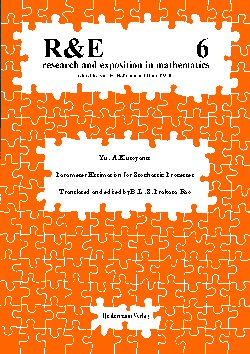R&E 6 Catalogue of allBook Series List of Booksin this Series Previous Book Next Book Research and Exposition in Mathematics -- Volume 6Enlarged Picture Yu. A. Kutoyants Parameter Estimation for Stochastic Processes 214 p., soft cover, ISBN 3-88538-206-7, 1984, out of print The book begins with some elementary concepts from the theory of probability and mathematical statistics and in particular some notions of asymptotic theory of statistical inference. The later chapters deal with asymptotic properties of estimators for various types of continuous time processes. The approach to asymptotic theory used throughout the book is via the log-likelihood ratio process. Many examples illustrate the results. The approach to the study of asymptotic theory of maximum likelihood and related estimators via stochastic processes as adopted here is also of potiential use in other areas, for instance, nonlinear regression. The probabilist or the statistician interested in theoretical aspects will appreciate the fact that this book gives a comprehensive treatment of asymptotic theory of statistical inference for several types of continuous time processes. It will be of great interest to mathematicians doing reseach in this area but can also be used for one semester courses for graduate students. "... gives a comprehensive treatment of asymptotical theory of statistical inference for diffusion and Poisson type processes. It will be of great interest to mathematicians doing research in this area, but can also be used for courses for graduate students". (Statistics & Decisions) "... excellent book ...". (Statistics)